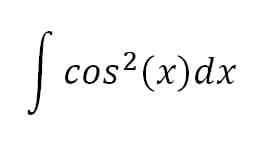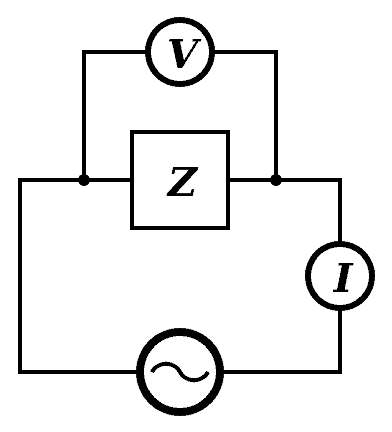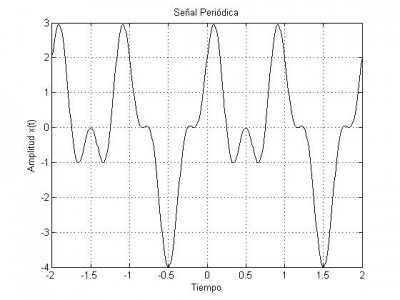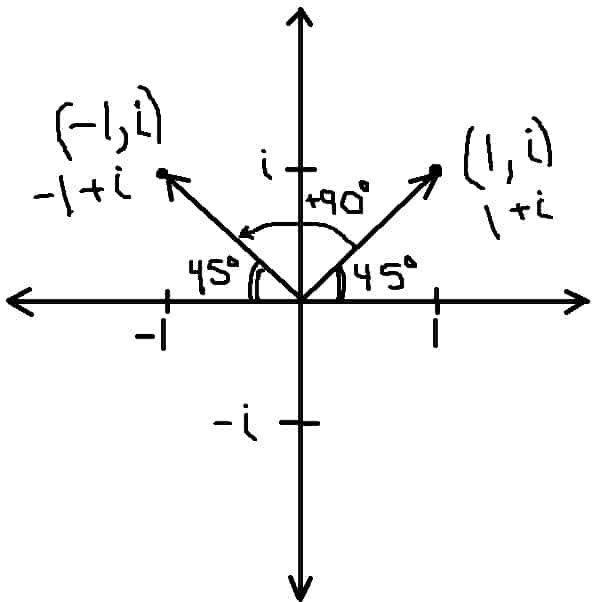# What Are Imaginary Numbers Used For? (7 Examples)

Imaginary numbers may not sound very useful, but they appear in a wide variety of topics in mathematics.  Not only are they useful in theory, but they have some applications in the math that makes some technologies possible.

So, what are imaginary numbers used for?  Imaginary numbers are a vital part of complex numbers, which are used in various topics including: evaluating integrals in calculus, second order differential equations, AC calculations in electricity, Fourier series, the Mandelbrot set, the quadratic formula, rotations, and vectors.

Of course, an imaginary number or a complex number is not a physical length or object that we can see.  Instead, it is an idea that can help us to understand other concepts better.

In this article, we’ll talk about what imaginary numbers are used for and take a closer look at 8 examples to show how diverse the applications are.

Let’s get started.

## What Are Imaginary Numbers Used For?

Imaginary numbers are necessary for complex numbers, which are used for a variety of applications, including:

• Calculus – evaluating some integrals
• Differential Equations – solution of second order differential equations.
• Electricity – calculations in AC (alternating current) electronics.
• Fourier series – used in signal processing for wireless technology.
• Mandelbrot Set – a fractal set of complex numbers that converges.
• Rotations & Vectors – representing 2D & 3D rotations.

Let’s take a look at each one of these in more detail, starting with calculus.

### Imaginary Numbers In Calculus

When evaluating integrals in calculus, it sometimes becomes difficult to find antiderivatives of trig functions (such as powers of sine and cosine).We can use Euler’s formula and imaginary numbers to find easier ways to evaluate some integrals that involve trig functions like sine and cosine.

Instead, we can use Euler’s formula to find equations for sin(x) and cos(x) in terms of exponentials.  Euler’s formula says that

• eix = cos(x) + isin(x)

If we substitute –x for x, this also implies that

• e-ix = cos(-x) + isin(-x)
• e-ix = cos(x) + isin(-x)  [cos(-x) = cos(x) since cos(x) is even]
• e-ix = cos(x) – isin(x)  [sin(-x) = -sin(x) since sin(x) is odd]

Adding the two equations eix = cos(x) + isin(x) and e-ix = cos(x) – isin(x) gives us:

• eix + e-ix = 2cos(x)
• (eix + e-ix) / 2 = cos(x)

Similarly, subtracting the two equations gives us:

• eix – e-ix = 2isin(x)
• (eix – e-ix) / 2i = sin(x)

We can then use these two identities to rewrite powers of sin(x) or cos(x) and evaluate integrals more easily.

### Imaginary Numbers In Differential Equations

When solving second order differential equations of the form

• ay’’ + by’ + cy = 0

we solve for roots of the characteristics equation

• at2 + bt + c = 0

In some cases, this will yield a pair of complex conjugate or imaginary roots.  Specifically, when the discriminant is negative, or b2 – 4ac < 0 (b2 < 4ac), we will get complex roots.

In all cases, we can express the roots r1 and r2 as complex numbers of the form c + di, where c and d are real numbers (d will be zero for real roots).

Remember that aside from the trivial solution y = 0, the solutions of this differential equation will have the form y = ert.

We can again use Euler’s formula to express these solutions in terms of sine and cosine functions.

### Imaginary Numbers In Electricity

When analyzing AC (alternating current) circuits, we can represent impedance as a complex number a + bi:

• Resistance is the real part a
• Reactance is the imaginary part bi

Note: in the context of electronics, j is often used instead of i to avoid confusion with I (which represents current).

In AC circuits, we use the following formula to relate voltage, current, and impedance (which has components of resistance and reactance):

• E = IZ

where E = voltage (sometimes V is used), I = current, and Z = impedance.In an AC circuit, V or E is voltage, I is current, and is impedance (which has resistance and reactance as components).

For example, if a circuit has voltage of 8 + 6j volts and impedance of 2 + 3j ohms, we can find the current as follows:

• E = IZ
• (8 + 6j) = I(2 + 3j)  [E = voltage = 8 + 6j and Z = impedance = 2 + 3j]
• (8 + 6j) / (2 + 3j) = I

To simplify, we multiply by the complex conjugate of the denominator on the top and bottom of the fraction on the left side of the equation.

The complex conjugate of 2 + 3j is 2 – 3j, so:

• (8 + 6j) / (2 + 3j)
• =(8 + 6j)(2 – 3j) / (2 + 3j)(2 – 3j)
• =(16 – 24j + 12j – 18j2) / (4 – 6j + 6j – 9j2)
• =(16 – 12j + 18) / (4 + 9)  [used j2 = -1 and combined like terms]
• =(34 – 12j) / 13
• =34/13 – (12/13)j

So, the current is 34/13 – (12/13)j.

### Imaginary Numbers In Fourier Series

A Fourier series (which rely on complex Fourier coefficients defined by integrals) uses the complex exponential einx.  We can use Fourier series for a Discrete Fourier Transform (DFT).

Basically, a Fourier transform can help us to “decompose” things into sine and cosine components.  For example, a sound wave can be written as a sum of two or more individual sine waves.Fourier series can help us to decompose things and write a wave as a sum of simpler sine waves.

Fourier transforms can be used in a broad range of applications, including:

• Circuit Design
• Crystallography
• Image Analysis, Compression, Filtering, & Reconstruction
• Signal Processing (such as wireless communications)
• Spectroscopy

### Imaginary Numbers In The Mandelbrot Set

The Mandelbrot Set is a famous set of complex numbers c for which fc(z) = z2 + c has a bounded modulus when iterated from z = 0.  This just means that the sequence { |fc(0)|, |fc(fc(0))|, … } has an upper limit for the values in the sequence.

Remember that |z| is the modulus of the complex number z, and if z = a + bi, then |z| = √(a2 + b2) is the distance from z to the origin (0, 0) in the complex plane.

### Imaginary Numbers From The Quadratic Formula

When solving a quadratic equation of the form

• ax2 + bx + c = 0

with real coefficients a, b, c, (a not equal to zero), we can solve using the quadratic formula, given by

The discriminant D = b2 – 4ac is the expression under the radical (the radicand) in the quadratic formula.  This value completely determines the nature of the solutions of the corresponding quadratic equation:

• D > 0 (b2 > 4ac): in this case, we have two distinct real solutions (real roots) for the quadratic equation.  The graph of this quadratic equation (a parabola) will intersect the x-axis twice.
• D = 0 (b2 = 4ac): in this case, we have one repeated real solution (a double root) for the quadratic equation.  The graph of this quadratic equation (a parabola) will intersect the x-axis once (the vertex of the parabola rests on the x-axis).
• D < 0 (b2 < 4ac): in this case, we have two complex conjugate solutions (complex roots) for the quadratic equation.  The graph of this quadratic equation (a parabola) will never intersect the x-axis (it will be always above or always below the x-axis).

The image below shows how the value of the discriminant tells us about the solutions of a quadratic equation.

When the discriminant is negative (D < 0), we are taking the square root of a negative in the quadratic formula.  The square root of a negative number will give us an imaginary number, and so we will get two complex (not real) solutions in this case.

If b is zero in the quadratic equation and a & c have the same sign, then we will get pure imaginary solutions (the real part of the two complex conjugates will be zero).

### Imaginary Numbers For Rotations

Since imaginary numbers can represent vectors in 2D or 3D space, we can also use them for rotations.  This is helpful in graphics for animation in making movies, video games, and simulations/training.

For example, let’s say we have the vector 1 + i in two-dimensional space.  This vector ends at a point that is a distance of √2 from the origin (0, 0), measured 45 degrees from the x-axis in the first quadrant.

If we multiply z = 1 + i by the complex number i = 0 + i, we get:

• iz
• =(0 + i)(1 + i)
• =0 + 0i + 1i + i2
• =-1 + i  [using i2 = -1]

This new vector ends at a point that is a distance of √2 from the origin (0, 0), measured 45 degrees from the x-axis in the second quadrant (135 degrees from the x-axis in the first quadrant).Multiplying by i is equivalent to a counterclockwise rotation by 90 degrees in the complex plane.

So, multiplying by i is equivalent to a counterclockwise rotation by 90 degrees.

Similarly, multiplying by –i is equivalent to a clockwise rotation by 90 degrees (or a counterclockwise rotation by 270 degrees).

Multiplying by -1 is equivalent to a rotation by 180 degrees.

Again, we can use Euler’s formula to make more sense of these rotations.  Since

• a + bi = reix = r(cos(x) + isin(x))

We can figure out which complex number to use for a given rotation by x (or, working backwards, figure out which angle we are rotating by when we multiply by a certain complex number).

For example, if we wish to rotate counterclockwise by 45 degrees (π/4), we would use the complex number:

• 1eiπ/4
• =1(cos(π/4) + isin(π/4))
• =1(√2/2 + i√2/2)
• =√2/2 + i√2/2

If we start with the complex number i = 0 + i (which lies on the circle with radius 1, at 90 degrees from the x-axis in the first quadrant), we can rotate counterclockwise by 45 degrees if we multiply by √2/2 + i√2/2:

• (0 + i)(√2/2 + i√2/2)
• =0 + 0 + i√2/2 + i2√2/2
• =-√2/2 + i√2/2

This is also a point on the circle with radius 1 (since the modulus or distance from the origin is 1), but it is rotated 45 degrees counterclockwise from 0 + i.

Note that if the modulus r = |z| of the complex number z is equal to 1, then multiplying a complex number by z will rotate the complex number, leaving it on the same circle in the complex plane.

However, if the modulus r = |z| of the complex number z is not equal to 1, then the length of the resulting product vector will change (in addition to the rotation that occurs).

If r > 1, the resulting vector becomes longer, and if r < 1, the resulting vector becomes shorter.

This article by Jacob Bell on Medium dives a little deeper into how to use imaginary numbers for rotations in the context of video games.

## Conclusion

Now that you know some uses of imaginary numbers, you may have an interest in learning more about them.